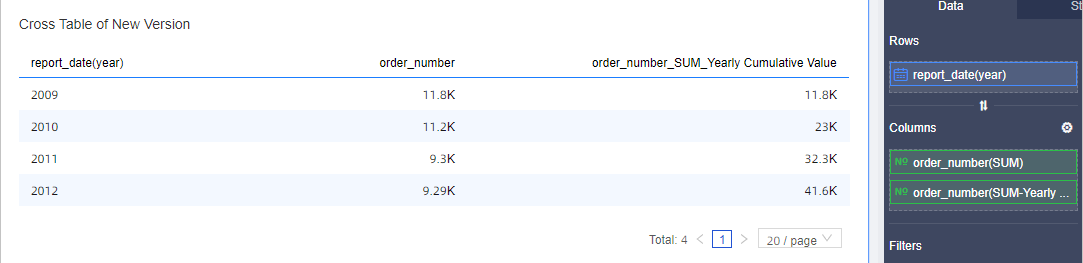This topic describes how to configure the cumulative calculation feature for a measure field.

## Background information

Cumulative Calculation calculates the sum of data from the initial date to the current date.

Date fields support the following cumulative calculation methods:
• Calculation methods for a date field in the yyyymmdd format
• Monthly Cumulative Value: You can calculate the data from the first day of the current month to the current day, such as from May 1, 2021 to May 29, 2021.
• Quarterly Cumulative Value: You can calculate the data from the first day of the current quarter to the current day, such as from April 1, 2021 to May 29, 2021.
• Current Year Cumulative Value: You can calculate the data from the first day of the current year to the current day, such as from January 1, 2021 to May 29, 2021.
• Yearly Cumulative Value: You can calculate the data from the first day of a specific year to the current day, such as from January 1, 2013 to May 29, 2021.
• Calculation methods for a date field in the yyyy-ww format
• Current Year Cumulative Value: You can calculate the data from the first week of the current year to the current week, such as from the first week of 2021 to the twenty-first week of 2021.
• Yearly Cumulative Value: You can calculate the data from the first week of a specific year to the current week, such as from the first week of 2013 to the twenty-first week of 2021.
• Calculation methods for a date field in the yyyymm format
• Quarterly Cumulative Value: You can calculate the data from the first month of the current quarter to the current month, such as from April 2021 to May 2021.
• Current Year Cumulative Value: You can calculate the data from the first month of the current year to the current month, such as from January 2021 to May 2021.
• Yearly Cumulative Value: You can calculate the data from the first month of a specific year to the current month, such as from January 2013 to May 2021.
• Calculation methods for a date field in the yyyyqq format
• Current Year Cumulative Value: You can calculate the data from the first quarter of the current year to the current quarter, such as from the first quarter of 2021 to the second quarter of 2021.
• Yearly Cumulative Value: You can calculate the data from the first quarter of a specific year to the current quarter, such as from the first quarter of 2013 to the second quarter of 2021.
• Calculation methods for a date field in the yyyy format
• Yearly Cumulative Value: You can calculate the data from the first year of a specific date to the current year, such as 2013 to 2021.
• A date field in the hh, hh:mm, hh:mm:ss, or yyyymmdd hh:mm:ss format does not support the cumulative calculation feature.
Field type Example Cumulative calculation
Year (in the format of yyyy) report_date(year)
• Yearly Cumulative Value
Quarter (in the format of yyyyqq) report_date(quarter)
• Current Year Cumulative Value
• Yearly Cumulative Value
Month (in the format of yyyymm) report_date(month)
• Quarterly Cumulative Value
• Current Year Cumulative Value
• Yearly Cumulative Value
Week (in the format of yyyy-ww) report_date(week)
• Current Year Cumulative Value
• Yearly Cumulative Value
Day (in the format of yyyymmdd) report_date(day)
• Monthly Cumulative Value
• Quarterly Cumulative Value
• Current Year Cumulative Value
• Yearly Cumulative Value
Hour (in the format of hh), Minute (in the format of hh:mm), Second (in the format of hh:mm:ss), and date field in the format of yyyymmdd hh:mm:ss report_date(hour), report_date(minute), report_date(second), report_date(ymdhms) N/A

## Limits

• The Comparison feature is not enabled for measure fields.
• Cumulative Calculation is supported only for fields marked with SUM (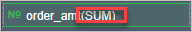).
• Cumulative Calculation is supported if the dimension field contains only one date field and its time granularity is year, quarter, month, week, or day.

## report_date(day)

This example shows how to configure Cumulative Calculation for fields in a cross table.

1. On the Data tab of the Chart Design pane, drag the report_date(day) field to Rows and drag five order_amt fields to Columns.
2. Move the pointer over order_amt, click the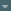icon on the right, and then choose Advanced Calculation > Cumulative Calculation > Monthly Cumulative Value.
You can perform the preceding operations to set Cumulative Calculation for other order_amt fields to Monthly Cumulative Value, Quarterly Cumulative Value, Current Year Cumulative Value, or Yearly Cumulative Value.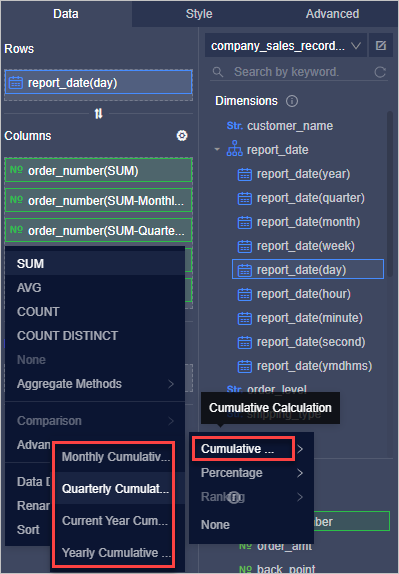3. Click Update.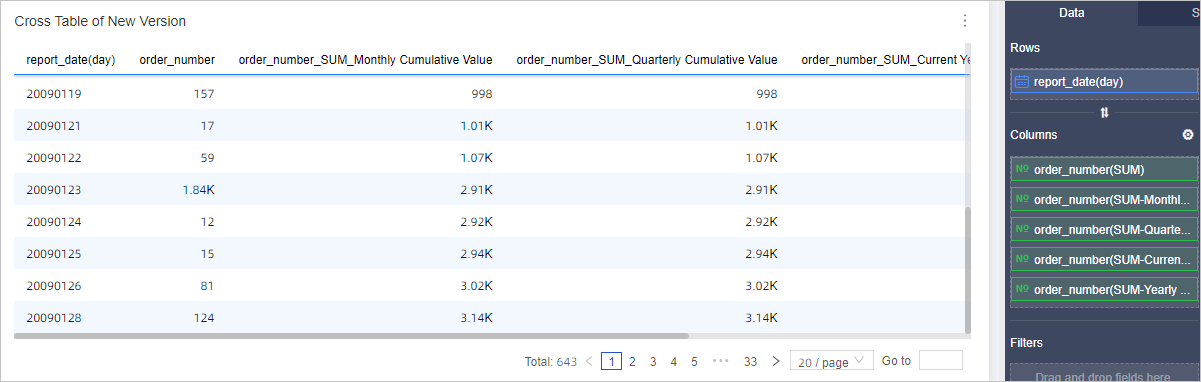## report_date(month)

This example shows how to configure Cumulative Calculation for fields in a cross table.

1. On the Data tab of the Chart Design pane, drag the report_date(month) field to Rows and drag four order_amt fields to Columns.
2. Move the pointer over order_amt, click theicon on the right, and then choose Advanced Calculation > Cumulative Calculation > Quarterly Cumulative Value.
You can perform the preceding operations to set Cumulative Calculation for other order_amt fields to Quarterly Cumulative Value, Current Year Cumulative Value, or Yearly Cumulative Value.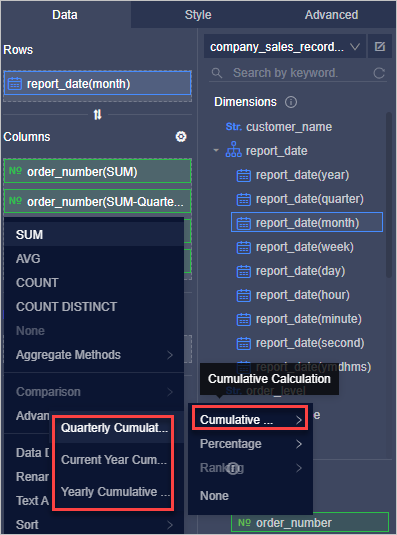3. Click Update.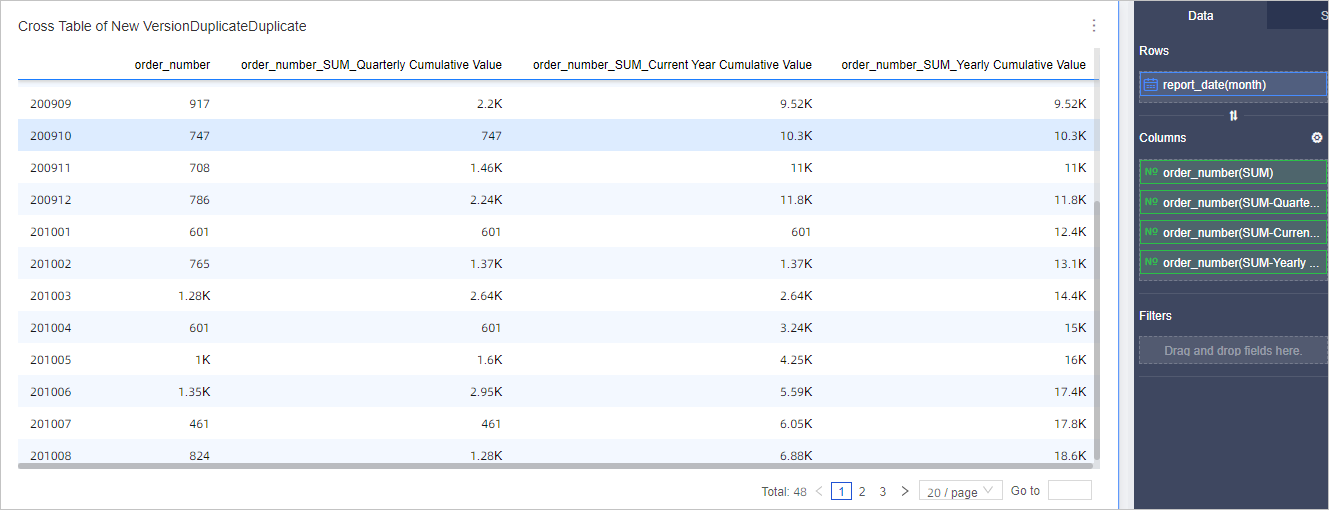## report_date(quarter)

This example shows how to configure Cumulative Calculation for fields in a cross table.

1. On the Data tab of the Chart Design pane, drag the report_date(quarter) field to Rows and drag three order_amt fields to Columns.
2. Move the pointer over order_amt, click theicon on the right, and then choose Advanced Calculation > Cumulative Calculation > Current Year Cumulative Value.
You can perform the preceding operations to set Cumulative Calculation for other order_amt fields to Current Year Cumulative Value or Yearly Cumulative Value.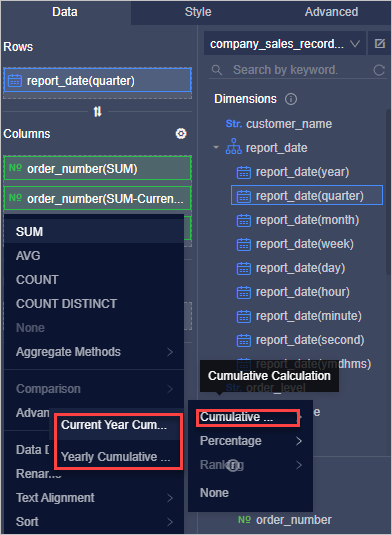3. Click Update.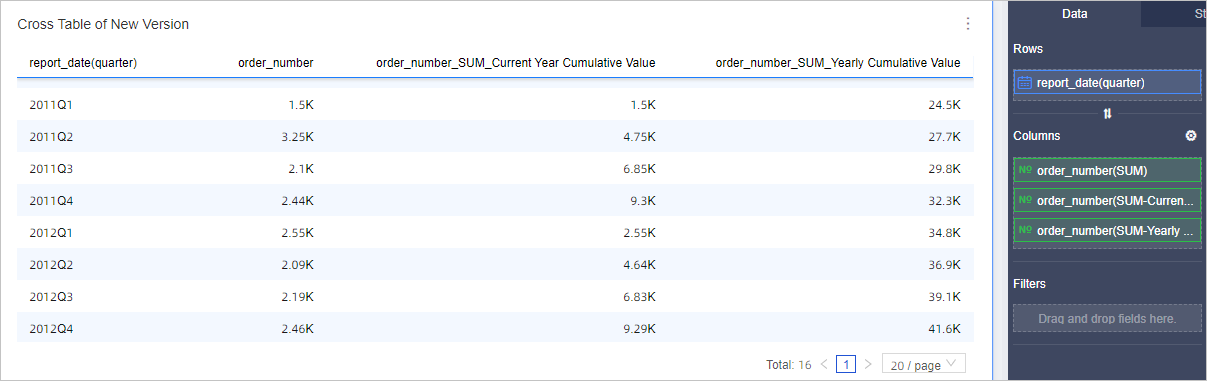## report_date(year)

This example shows how to configure Cumulative Calculation for fields in a cross table.

1. On the Data tab of the Chart Design pane, drag the report_date(year) field to Rows and drag two order_amt fields to Columns.
2. Move the pointer over order_amt, click theicon on the right, and then choose Advanced Calculation > Cumulative Calculation > Yearly Cumulative Value.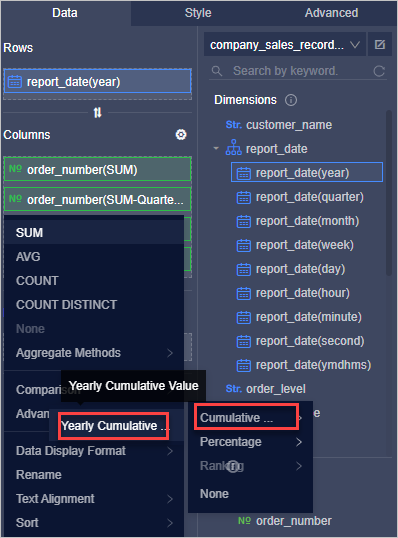3. Click Update.